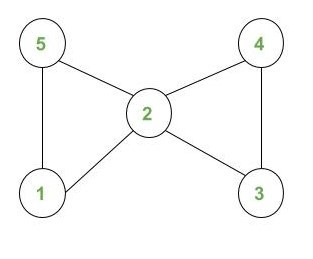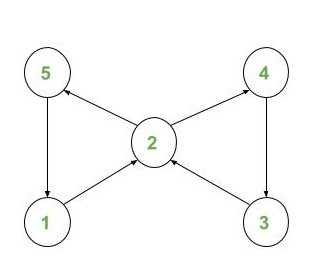# Conversion of an Undirected Graph to a Directed Euler Circuit

Given an undirected graph with V nodes (say numbered from 1 to V) and E edges, the task is to check whether the graph is an Euler Graph or not and if so then convert it into a Directed Euler Circuit.

A Directed Euler Circuit is a directed graph such that if you start traversing the graph from any node and travel through each edge exactly once you will end up on the starting node.

Note: While traversing a Euler circuit every edge is traversed exactly once. A node can be traversed more than once if needed but an edge cannot be traversed more than once.

Example:

Input:Output:
1 2
2 5
5 1
2 4
4 3
3 2
Explanation:
The Directed Euler Circuit for the given undirected graph will be:## Recommended: Please try your approach on {IDE} first, before moving on to the solution.

Approach:

1. First, we need to make sure the given Undirected Graph is Eulerian or not. If the undirected graph is not Eulerian we cannot convert it to a Directed Eulerian Graph.

• To check it we just need to calculate the degree of every node. If the degree of all nodes is even and not equal to 0 then the graph is Eulerian.
2. We will be using Depth First Search Traversal to assign the directions.

• While traversing we will set the direction of an edge from parent to child. We will maintain a map to make sure an edge is traversed only once.

Below is the implementation of the above algorithm:

 `// C++ program to Convert an ` `// Undirected Graph to a ` `// Directed Euler Circuit ` ` `  `#include ` `using` `namespace` `std; ` ` `  `vector<``int``> g; ` ` `  `// Array to store degree ` `// of nodes. ` `int` `deg = { 0 }; ` ` `  `// Map to keep a track of ` `// visited edges ` `map, ``int``> m1; ` ` `  `// Vector to store the edge ` `// pairs ` `vector > v; ` ` `  `// Function to add Edge ` `void` `addEdge(``int` `u, ``int` `v) ` `{ ` `    ``deg[u]++; ` `    ``deg[v]++; ` `    ``g[u].push_back(v); ` `    ``g[v].push_back(u); ` `} ` ` `  `// Function to check if graph ` `// is Eulerian or not ` `bool` `CheckEulerian(``int` `n) ` `{ ` `    ``int` `check = 0; ` `    ``for` `(``int` `i = 1; i <= n; i++) { ` `        ``// Checking if odd degree ` `        ``// or zero degree nodes ` `        ``// are present ` `        ``if` `(deg[i] % 2 || deg[i] == 0) { ` `            ``check = 1; ` `            ``break``; ` `        ``} ` `    ``} ` ` `  `    ``// If any degree is odd or ` `    ``// any vertex has degree 0 ` `    ``if` `(check) { ` `        ``return` `false``; ` `    ``} ` `    ``return` `true``; ` `} ` `// DFS Function to assign the direction ` `void` `DirectedEuler(``int` `node, ` `                   ``vector<``int``> g[]) ` `{ ` `    ``for` `(``auto` `i = g[node].begin(); ` `         ``i != g[node].end(); i++) { ` `        ``// Checking if edge is already ` `        ``// visited ` `        ``if` `(m1[make_pair(node, *i)] ` `            ``|| m1[make_pair(*i, node)]) ` `            ``continue``; ` ` `  `        ``m1[make_pair(node, *i)]++; ` ` `  `        ``// Storing the edge ` `        ``v.push_back(make_pair(node, *i)); ` `        ``DirectedEuler(*i, g); ` `    ``} ` `} ` ` `  `// Function prints the convert ` `// Directed graph ` `void` `ConvertDirectedEuler(``int` `n, ` `                          ``int` `e) ` `{ ` `    ``if` `(!CheckEulerian(n)) { ` `        ``cout << ``"NOT POSSIBLE"` `             ``<< endl; ` `        ``return``; ` `    ``} ` ` `  `    ``DirectedEuler(1, g); ` ` `  `    ``// Printing directed edges ` `    ``for` `(``auto` `i = v.begin(); ` `         ``i != v.end(); i++) { ` `        ``cout << (*i).first ` `             ``<< ``" "` `             ``<< (*i).second ` `             ``<< endl; ` `    ``} ` `} ` `// Driver code ` `int` `main() ` `{ ` `    ``int` `N = 5; ` `    ``int` `E = 6; ` `    ``addEdge(1, 2); ` `    ``addEdge(1, 5); ` `    ``addEdge(5, 2); ` `    ``addEdge(2, 4); ` `    ``addEdge(2, 3); ` `    ``addEdge(4, 3); ` ` `  `    ``ConvertDirectedEuler(N, E); ` `} `

Output:

```1 2
2 5
5 1
2 4
4 3
3 2
```

Time Complexity: O(( V + E ) * log( E ))
Space Complexity: O(max( V, E ))

Don’t stop now and take your learning to the next level. Learn all the important concepts of Data Structures and Algorithms with the help of the most trusted course: DSA Self Paced. Become industry ready at a student-friendly price.

My Personal Notes arrow_drop_upCheck out this Author's contributed articles.

If you like GeeksforGeeks and would like to contribute, you can also write an article using contribute.geeksforgeeks.org or mail your article to contribute@geeksforgeeks.org. See your article appearing on the GeeksforGeeks main page and help other Geeks.

Please Improve this article if you find anything incorrect by clicking on the "Improve Article" button below.